You are currently offline. Some features of the site may not work correctly.

# Journal of Approximation Theory

The Journal of Approximation Theory is "devoted to advances in pure and applied approximation theory and related areas."
Wikipedia

## Papers overview

Semantic Scholar uses AI to extract papers important to this topic.
2017
2017
It is shown that two conditions $f(a + \cdot) - f(\cdot) \in L^p(R)$, and $(\sin b \cdot) f(\cdot) \in L^p(R)$ guarantee \$f \in L… Expand
2015
2015
• J. Approx. Theory
• 2015
• Corpus ID: 14428063
Let E be a compact set of positive logarithmic capacity in the complex plane and let { P n ( z ) } 1 ∞ be a sequence of… Expand
•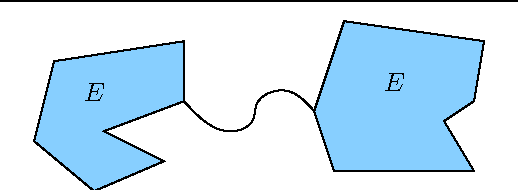•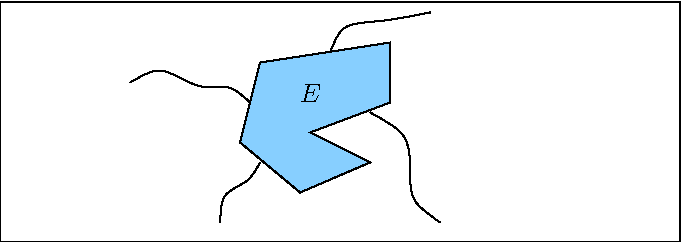•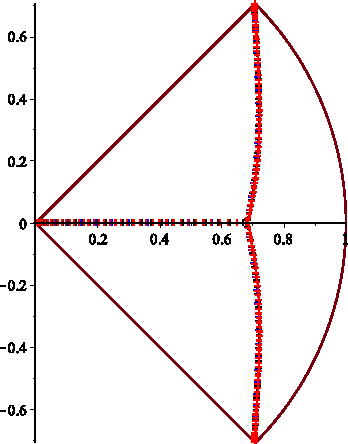•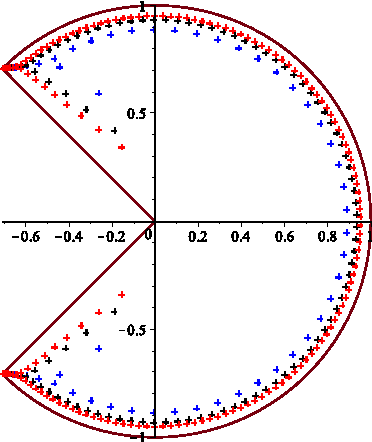2015
2015
• J. Approx. Theory
• 2015
• Corpus ID: 21680369
We study the inequality between the uniform norm of the derivative of an algebraic polynomial of degree n and the integral norm… Expand
2012
2012
• J. Approx. Theory
• 2012
• Corpus ID: 3049352
We present results on interpolation and L"1-approximation of periodic functions by trigonometric polynomials and trigonometric… Expand
2010
2010
• S. Dekel
• J. Approx. Theory
• 2010
• Corpus ID: 205705723
It is well known that for any bounded Lipschitz graph domain Ω⊂Rd, r≥1 and 1≤p≤∞ there exist constants C1(d,r),C2(Ω,d,r,p)>0 such… Expand
2007
2007
• J. Approx. Theory
• 2007
• Corpus ID: 27697931
The method developed in Duran and Grunbaum [Orthogonal matrix polynomials satisfying second order differential equations… Expand
2007
2007
• J. Approx. Theory
• 2007
• Corpus ID: 482713
In this paper we investigate the L"2-solutions of vector refinement equations with exponentially decaying masks and a general… Expand
2004
2004
• J. Approx. Theory
• 2004
• Corpus ID: 1222685
Recently Denisov (aka Dennisov) (Proc. Amer. Math. Soc.) has proved the following remarkable extension of Rakhmanov's theorem… Expand
2003
2003
Cette these porte sur la restauration des signaux bruites observes sur des plans d'experience aleatoires. Trois methodes sont… Expand
•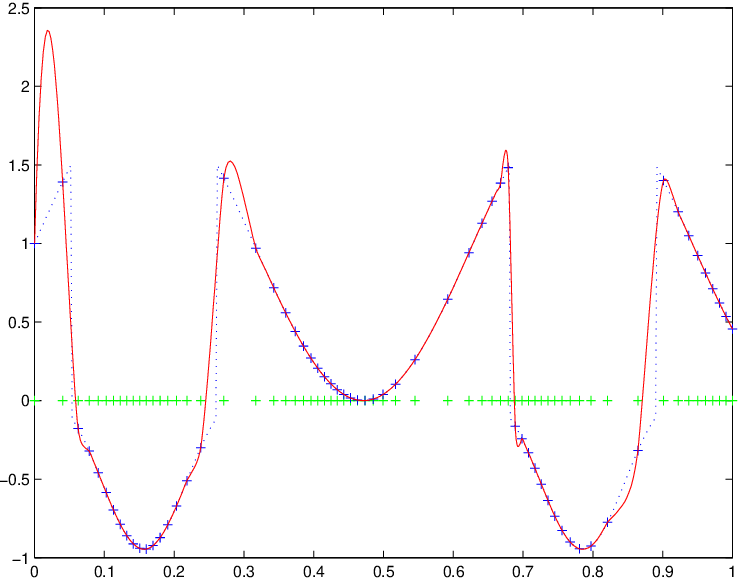•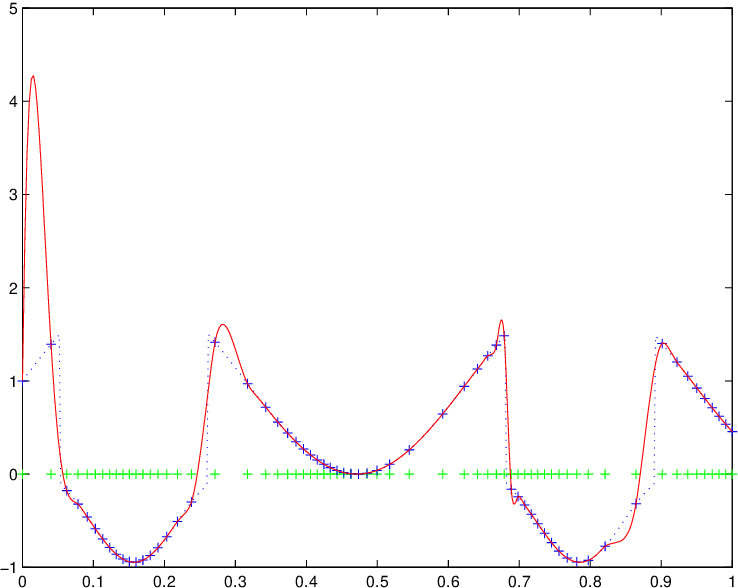•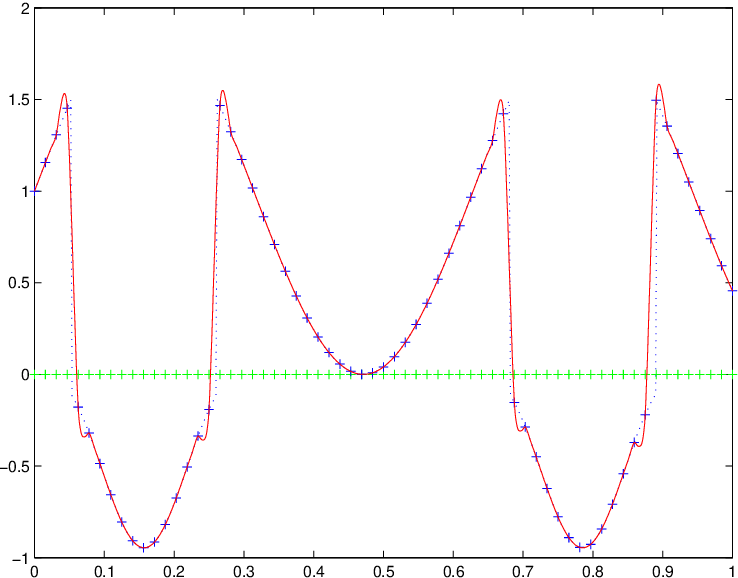•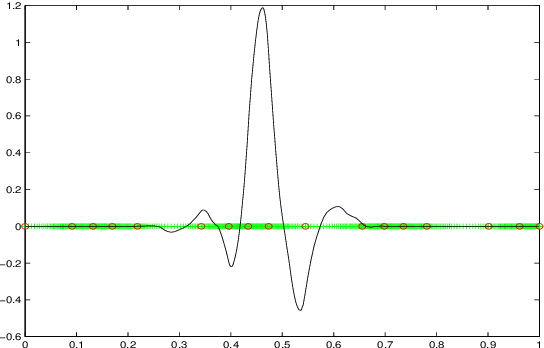•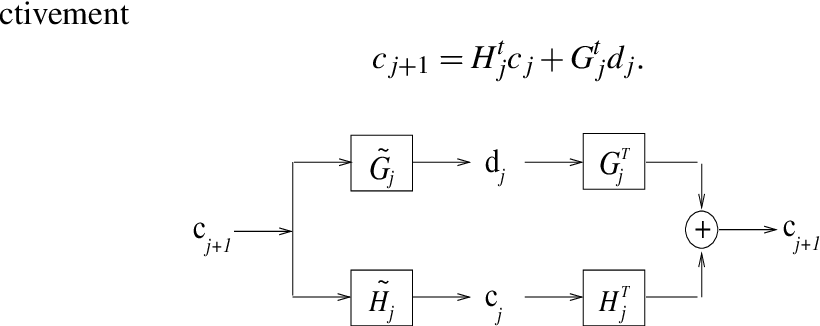2003
2003
• J. Approx. Theory
• 2003
• Corpus ID: 2835087
We prove that the weak dual greedy algorithm converges in any subspace of a quotient of Lp when 1 < p < ∞.